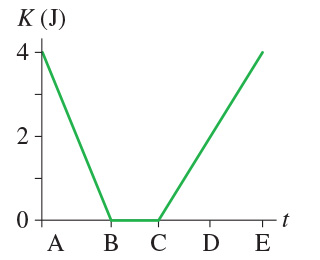# (figure 1) is the kinetic-energy graph for a 2.0 kg object moving along the x-axis.(Figure 1) is the kinetic-energy graph for a 2.0 kg object
moving along the x-axis. x Part A Part A Determine the work done on the object during each of the four
intervals AB, BC,
CD, and DE. A B B C C D D E Express your answers using one significant figure. Enter your
answers numerically separated by a comma.

Determine the work done on the object during each of the four
intervals AB, BC, CD, and DE.
Express your answers using one significant figure. Enter your
answers numerically separated by a comma.
Work is the transfer of energy through a force.
AB = 0 – 4 = -4 J
BC = 0 – 0 = 0 J
CD = 2 – 0 = 2 J
DE = 4 – 2 = 2 J